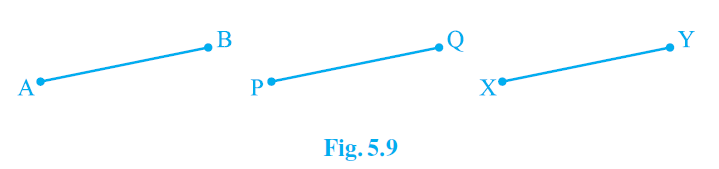Newbie

# Which of the following statements are true and which are false? Give reasons for your answers. (i) Only one line can pass through a single point. (ii) There are an infinite number of lines which pass through two distinct points. (iii) A terminated line can be produced indefinitely on both the sides. (iv) If two circles are equal, then their radii are equal. (v) In Fig. 5.9, if AB = PQ and PQ = XY, then AB = XY. Q.1

• 0

Find this important question of ncert class 9th of chapter Introduction To Euclid’s Geometry   .Sir please help me to find out the easiest and simplest solution of question 1 of exercise 5.1, give me the best solution of this question. Give me the best solution of this question.Which of the following statements are true and which are false? Give reasons for your answers. (i) Only one line can pass through a single point. (ii) There are an infinite number of lines which pass through two distinct points. (iii) A terminated line can be produced indefinitely on both the sides. (iv) If two circles are equal, then their radii are equal. (v) In Fig. 5.9, if AB = PQ and PQ = XY, then AB = XY.

Share

1.Solution:

(i) False

There can be infinite number of lines that can be drawn through a single point. Hence, the statement mentioned is False

(ii) False

Through two distinct points there can be only one line that can be drawn. Hence, the statement mentioned is False

(iii) True

A line that is terminated can be indefinitely produced on both sides as a line can be extended on both its sides infinitely. Hence, the statement mentioned is True.

(iv) True

The radii of two circles are equal when the two circles are equal. The circumference and the centre of both the circles coincide; and thus, the radius of the two circles should be equal. Hence, the statement mentioned is True.

(v) True

According to Euclid’s 1st axiom- “Things which are equal to the same thing are also equal to one another”. Hence, the statement mentioned is True.

• 0